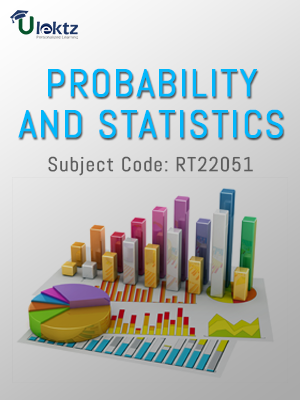The site would be on maintenance from 8th Jul 2019 2.00pm to 8:00pm. You may experience some issue during this time.uLektz apps

•My WalletMy Order
•My Profile
•My Connections
•My Books
•My Videos
•My Tests
•My Calender
•My Messages
•My Shopping Cart
•My Orders
•Account Settings
•Help

uLektz apps

Book DetailsProbability And Statistics

Course Code:RT22051

Author:uLektz

University:

Regulation:2013

Categories:Engineering Mathematics

Format :ePUB3 (DRM Protected)

Type :eBook

FREE

Description :Probability And Statistics of RT22051 covers the latest syllabus prescribed by JNTU Kakinada for regulation 2013. Author: uLektz, Published by uLektz Learning Solutions Private Limited.

Note : No printed book. Only ebook. Access eBook using uLektz apps for Android, iOS and Windows Desktop PC.

Topics
UNIT I RANDOM VARIABLES AND DISTRIBUTIONS

1.1 Introduction- Random variables-Distribution function-Discrete distributions ( Review of Binomial and Poisson distributions)

1.2 Continuous distributions: Normal, Normal approximation to Binomial distribution, Gamma and Weibull distributions

UNIT II Moments and Generating functions

2.1 Introduction-Mathematical expectation and properties

2.2 Moment generating function-Moments of standard distributions ( Binomial, Poisson and Normal distributions) – Properties

UNIT III SAMPLING THEORY

3.1 Introduction - Population and samples

3.2 Sampling distribution of mean for large and small samples (with known and unknown variance)

3.3 Proportion sums and differences of means-Sampling distribution of variance -Point and interval estimators for means and proportions

UNIT IV TESTS OF HYPOTHESIS

4.1 Introduction - Type I and Type II errors

4.2 Maximum error - One tail, two-tail tests

4.3 Tests concerning one mean and proportion

4.4 Two means- Proportions and their differences using Z-test, Student’s t-test - F-test and Chi -square test

4.5 ANOVA for one-way and two-way classified data

UNIT V CURVE FITTING AND CORRELATION

5.1 Introduction - Fitting a straight line

5.2 Second degree curve-exponential curve-power curve by method of least squares

5.3 Simple Correlation and Regression

5.4 Rank correlation - Multiple regression

UNIT VI STATISTICAL QUALITY CONTROL METHODS

6.1 Introduction - Methods for preparing control charts

6.2 Problems using x-bar, p, R charts and attribute charts

Related Books